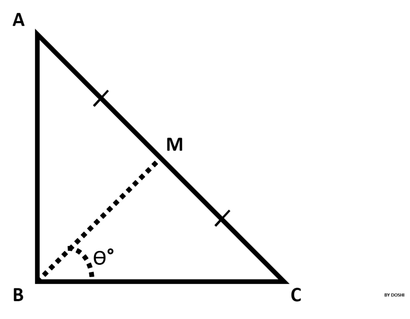# Find Angle MBC - Python HackerRank Solution

## Find Angle MBC - Python HackerRank Solution

Hello Friends, How are you? Today I am going to solve the HackerRank Python Find Angle MBC Problem with a very easy explanation. In this article, you will get more than one approach to solve this problem. So let's start-

### HackerRank Python Find Angle MBC Solution - Problem StatementSource: Find Angle MBC - Python HackerRank

ABC is a right triangle, 90° at B.
Therefore, angle ABC = 90°.
Point M is the midpoint of hypotenuse AC.

You are given the lengths AB and BC.

Input Format

The first line contains the length of side AB.
The second line contains the length of side BC.

Constraints

0 < AB <= 100
0 < BC <= 100
Lengths AB and BC are natural numbers.

Output Format

Output angle MBC in degrees.
Note: Round the angle to the nearest integer.

Examples:

If the angle is 56.5000001°, then output 57°.
If the angle is 56.5000000°, then output 57°.
If the angle is 56.4999999°, then output 56°.

Sample Input 0

10 10 {codeBox}

Sample Output 0

45° {codeBox}

### Python Find Angle MBC - Hacker Rank Solution

Approach I: Find Angle MBC HackerRank Python Solution

``````# ========================
#       Information
# ========================

# Name: Find Angle MBC - Python HackerRank Problem
# Difficulty: Medium
# Max Score: 10
# Language: Python 3

# ========================
#         Solution Start
# ========================

# Find Angle MBC Python - Hacker Rank Solution

import math
ab=int(input())
bc=int(input())
ca=math.hypot(ab,bc)
mc=ca/2
bca=math.asin(1*ab/ca)
bm=math.sqrt((bc**2+mc**2)-(2*bc*mc*math.cos(bca)))
mbc=math.asin(math.sin(bca)*mc/bm)
print(int(round(math.degrees(mbc),0)),'\u00B0',sep='')

# Find Angle MBC in Python - Hacker Rank Solution END
# MyEduWaves``````

Approach II: Find Angle MBC HackerRank Python Solution

``````# ========================
#       Information
# ========================

# Name: Find Angle MBC - Python HackerRank Problem
# Difficulty: Medium
# Max Score: 10
# Language: Python 3

# ========================
#         Solution Start
# ========================

# Find Angle MBC Python - Hacker Rank Solution

import math

ab = input()
bc = input()

h = math.sqrt(ab**2 + bc**2)
h = h / 2.0